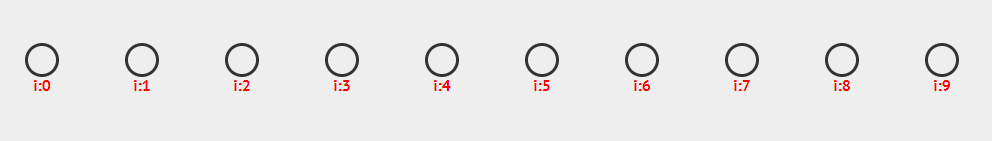# 数据结构 - 散列表

## Hash Table

ZingLix August 20, 2017

## 一、基本思想## 二、散列函数

### 除法散列法

$h(k)=k\ mod\ m$

### 乘法散列法

$h(k)=\lfloor m(kA\ mod\ 1)\rfloor$

## 三、解决冲突

### 分离链接法#### 装载因子

$\alpha = \frac{n}{m}$

### 开放寻址法

#### 线性探查

$h(k,i)=(h'(k)+i)\ mod\ m,\ \ \ \ i=0,1,\cdots,m-1$#### 二次探查

$h(k,i)=(h'(k)+c_1 i+c_2 i^2 )\ mod\ m$

#### 双重散列

$h(k,i)=(h_1(k)+ih_2(k))\ mod\ m$

$h_1(k) = k\ mod\ n , h_2(k) = 1 + (k\ mod\ m')$